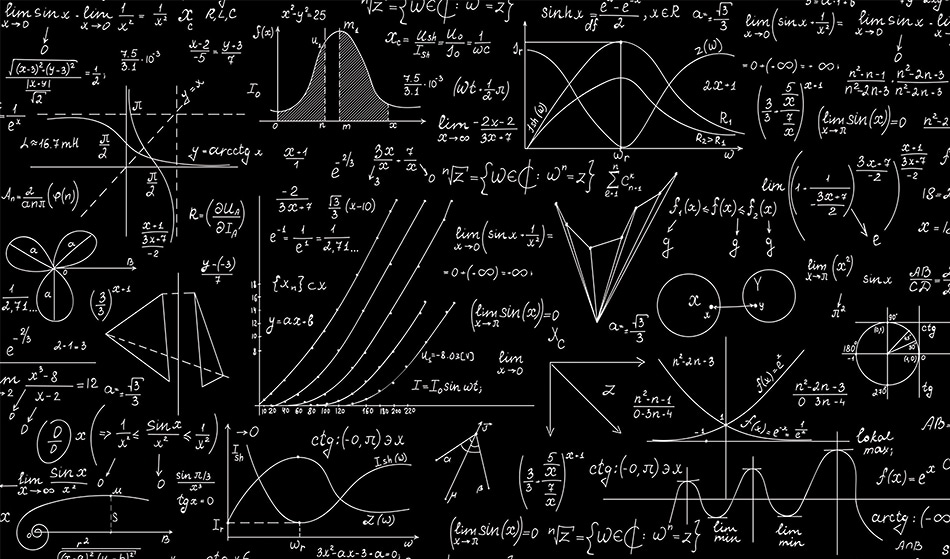# A Fractional Approach to Calculus of VariationsMarina Sun / Shutterstock

In physics, according to the variation principle, the path taken by a particle between two points is an extremum of the action integral. This principle provides information about several symmetries of a system and their corresponding conserved quantities.

It is also possible to formulate all fundamental theories of physics (such as classical physics, quantum physics, general relativity and quantum field theories) with this principle. It is advantageous in many respects to describe the nature of the variation approach.

Variation techniques cannot be applied directly to non-conservative systems. According to Bauer’s theorem, there is no single linear dissipative equation of motion with constant coefficients using variation principle techniques. However, in this theorem, the orders of derivatives are integers.

Non-conservative systems can be described with the variation principle by using the fractional calculus. This was first proved by Riewe, and his study can be regarded as the beginning of the fractional calculus of variations. Many researchers have since developed different versions of this new technique and made significant contributions.

Fractional calculus is a branch of mathematics that generalizes the derivative (and integrals) of a function to a non-integer. Its origins go back more than 300 years. The history of fractional calculus is as old as calculus itself. In 1695 in a letter to L’ Hospital, Leibniz looked into the meaning of , if    . “This is an apparent paradox from which one-day useful consequence will be drawn.” Leibniz replied, and fractional calculus was born.

However, in fractional calculus, all derivatives are non-local operators. Therefore, fractional operators lead us to take non-local effects into account. In the time coordinate, non-local effects manifest as the memory effect, which states that the actual behavior of a given object is only influenced by events that happened in the past. For this reason, fractional mathematical physics approaches lead to a more effective way to investigate the problems in non-linear and complex systems.

In the last few decades, fractional calculus has appeared in different fields of science, including electrical circuits, quantum mechanics, statistical physics, and nuclear physics. Consequently, according to fractional calculus, physical processes evolve in fractal space-time. Hence, this behavior of space and time is not compatible with constraints of Euclidean geometry and context of Markovian approach. Therefore, using the fractional calculus instead of standard mathematics allows us to make a more realistic description of nature

On the other hand, some difficulties are encountered in this formalism. The most important difficulty is the simultaneous presence of left and right derivatives. From the viewpoint of physics, in the time domain, the left fractional derivative represents the past state of the dynamical process, whereas the right fractional derivative represents the future state of the dynamical process. In other words, the present state of any process depends on the past and future states of the process in the fractional approach.

However, this means that the fractional version of Euler-Lagrange equation clearly violates the principle of causality for physics. In physics, the principle of causality states that the present behavior of a given object is only influenced by all its past states.

Nevertheless, in quantum mechanics, according to Feynman’s and Stückelberg’s views, antiparticles propagate backwards in time and hence fractional right derivative operator could be used to describe the velocity of an antiparticle. However, presence of right derivative seems to be a definitive failure for the non-conservative systems. In the literature, there are several techniques which are proposed to overcome this problem.

## Sources

• P. S. Bauer, Proceedings of the National Academy of Sciences of the United States of America, 17, 311 (1931)
• F. Riewe, Physical Review E 53, 2, 1890 (1996)
• F. Riewe, Physical Review E 55, 3, 3581 (1997)
• R. P. Feynman, Physical Review 76, 6, 749 (1949)
• E.C.G Stueckelberg, Helvetica Physica Acta 14, 322 (1941)
• E.C.G Stueckelberg, Helvetica Physica Acta 15, 23 (1942)

Disclaimer: The views expressed here are those of the author expressed in their private capacity and do not necessarily represent the views of AZoM.com Limited T/A AZoNetwork the owner and operator of this website. This disclaimer forms part of the Terms and conditions of use of this website.Written by

### Kazim Gokhan Atman

Kazim Gokhan Atman is a Ph.D. student in mathematical physics. He is mainly focused on fractional calculus and possible violation of locality at a small distance in Quantum Field Theory.

## Citations

• APA

Atman, Kazim. (2019, July 17). A Fractional Approach to Calculus of Variations. AZoQuantum. Retrieved on October 31, 2020 from https://www.azoquantum.com/Article.aspx?ArticleID=120.

• MLA

Atman, Kazim. "A Fractional Approach to Calculus of Variations". AZoQuantum. 31 October 2020. <https://www.azoquantum.com/Article.aspx?ArticleID=120>.

• Chicago

Atman, Kazim. "A Fractional Approach to Calculus of Variations". AZoQuantum. https://www.azoquantum.com/Article.aspx?ArticleID=120. (accessed October 31, 2020).

• Harvard

Atman, Kazim. 2019. A Fractional Approach to Calculus of Variations. AZoQuantum, viewed 31 October 2020, https://www.azoquantum.com/Article.aspx?ArticleID=120.

## Tell Us What You Think